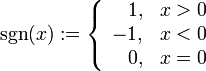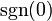# Difference between revisions of "Signum function"

This article is about a particular function from a subset of the real numbers to the real numbers. Information about the function, including its domain, range, and key data relating to graphing, differentiation, and integration, is presented in the article.
View a complete list of particular functions on this wiki

## Definition

The signum function, denoted$\operatorname{sgn}$, is defined as follows:$\operatorname{sgn}(x) := \left\lbrace \begin{array}{rl} 1, & x > 0 \\ -1, & x < 0 \\ 0, & x = 0 \\\end{array}\right.$

Note: In the definition given here, we define the value$\operatorname{sgn}(0)$ to be zero. In some alternative definitions,$\operatorname{sgn}(0)$ is considered to be undefined, i.e., the domain of$\operatorname{sgn}$ is taken to be the nonzero reals.